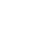# HDOJ2037：今年暑假不AC

Time Limit: 2000/1000 MS (Java/Others) Memory Limit: 65536/32768 K (Java/Others)
Total Submission(s): 27554 Accepted Submission(s): 14558
Problem Description
“今年暑假不AC？”
“是的。”
“那你干什么呢？”
“看世界杯呀，笨蛋！”
“@#\$%^&*%…”

Input

Output

Sample Input
12
1 3
3 4
0 7
3 8
15 19
15 20
10 15
8 18
6 12
5 10
4 14
2 9
0

Sample Output
5

```#include
int main()
{
int t,num,n,ni,nii,time,s;
scanf("%d",&n);
for(ni=0;ni

#include
int main()
{
int st, end;
int n, a, b;
while (scanf("%d", &n) && n != 0)
{
for (int i = 0; iend[j])按后面排好  交换
if (end[i]>end[j])
{
a = end[i];
end[i] = end[j];
end[j] = a;
b = st[i];
st[i] = st[j];
st[j] = b;
// 再接着开始排列
}
}
int sum = 0, max = 0;
for (int i = 0; i= max)
{
sum++;
max = end[i];
}
printf("%dn", sum);
}
return 0;
}

#include
#include
#include
#define MAX 1000
using namespace std;
pair p[MAX];
int main()
{
int n;
int s, e;
while (scanf("%d", &n)>0 && n)
{
for (int i = 0; i

## 《HDOJ2037：今年暑假不AC》有1个想法

1.写码的柳絮 文章作者

第二段代码是WA代码。

回复